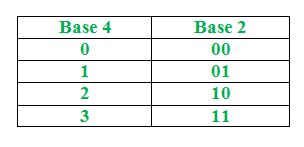GFG App
Open AppBrowser
Continue

# Python program to convert Base 4 system to binary number

Given a base 4 number N, the task is to write a python program to print its binary equivalent.

Conversion Table:Examples:

```Input       : N=11002233
Output      : 101000010101111
Explanation : From that conversion table we changed 1 to 01, 2 to 10 ,3 to 11 ,0 to 00.```
```Input : N=321321
Output: 111001111001```

Method 1:

• Take an empty string say resultstr.
• We convert the decimal number to string.
• Traverse the string and convert each character to an integer
• If the integer is 1 or 0 then before converting to binary add ‘0’ to resultstr (Because we cannot have 01,00 in integers)
• Now convert this integer to binary string and concatenate the resultant binary string to resultstr.
• Convert resultstr to integer(which removes leading zeros).
• Return resultstr.

Below is the implementation of the above approach as follows:

## Python3

 `# function which converts decimal to binary` `def` `decToBin(num):`   `    ``# Using default binary conversion functions` `    ``binary ``=` `bin``(num)`   `    ``# removing first two character as the` `    ``# result is always in the form 0bXXXXXXX` `    ``# by taking characters after index 2` `    ``binary ``=` `binary[``2``:]` `    ``return` `binary`   `# function to convert base4 to binary`     `def` `convert(num):`   `    ``# Taking a empty string` `    ``resultstr ``=` `""`   `    ``# converting number to string` `    ``numstring ``=` `str``(num)`   `    ``# Traversing string` `    ``for` `i ``in` `numstring:`   `        ``# converting this character to integer` `        ``i ``=` `int``(i)`   `        ``# if i is 1 or 0 then add '0' to result` `        ``# string` `        ``if``(i ``=``=` `1` `or` `i ``=``=` `0``):` `            ``resultstr ``=` `resultstr``+``'0'`   `        ``# passing this integer to get converted to` `        ``# binary` `        ``binary ``=` `decToBin(i)`   `        ``# print(binary)` `        ``# Concatenating this binary string to result` `        ``# string` `        ``resultstr ``=` `resultstr``+``binary`   `    ``# Converting resultstr to integer` `    ``resultstr ``=` `int``(resultstr)`   `    ``# Return result string` `    ``return` `resultstr`     `# Driver code` `Number ``=` `11002233`   `# Passing this number to convert function` `print``(convert(Number))`

Output:

`101000010101111`

Time Complexity: O(n), Auxiliary Space: O(1)

Here n is the length of the no

#### Method 2:

Step-by-step approach:

• The base4_to_binary function takes a string num representing a number in base 4 as input.
• The function first converts the number to base 10 by multiplying each digit in the number by 4 raised to the power of its position (i.e., the rightmost digit is in position 0, the next digit is in position 1, and so on). This is done by iterating through the digits of the number in reverse order and adding the product of the digit and 4 raised to the power of its position to a variable base10.
• The function then converts the number from base 10 to binary using the built-in bin() function in Python. The bin() function returns a string with a ‘0b’ prefix, so we slice off the first two characters to get the binary representation of the number as a string.
• The function returns the binary representation of the number as a string.

## Python3

 `def` `base4_to_binary(num):` `    ``# Convert the number to base 10` `    ``base10 ``=` `0` `    ``for` `i, digit ``in` `enumerate``(num[::``-``1``]):` `        ``base10 ``+``=` `int``(digit) ``*` `(``4` `*``*` `i)` `    `  `    ``# Convert the number to binary` `    ``binary ``=` `bin``(base10)[``2``:]` `    ``return` `binary` `num ``=` `"11002233"` `binary ``=` `base4_to_binary(num)` `print``(f``"The binary representation of {num} is {binary}"``)` `# Output: The binary representation of 1032 is 10011010`   `num ``=` `"321321"` `binary ``=` `base4_to_binary(num)` `print``(f``"The binary representation of {num} is {binary}"``)` `# Output: The binary representation of 30 is 11110`

Output

```The binary representation of 11002233 is 101000010101111
The binary representation of 321321 is 111001111001```

Time complexity: O(n)
Auxiliary Space: O(1)

My Personal Notes arrow_drop_up